# Airplane in Wind

The cross-country navigation of an aircraft involves the vector addition of relative velocities since the resultant ground speed is the vector sum of the airspeed and the wind velocity. Using the air as the intermediate reference frame, ground speed can be expressed as: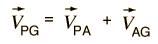The velocity of the plane with respect to the ground is equal to the velocity of the plane with respect to the air plus the velocity of the air with respect to the ground.### Calculation

Index

Relative velocity

 HyperPhysics***** Mechanics R Nave
Go Back

# Airplane in Wind: Calculation of Ground Velocity

Navigation directions are usually expressed in terms of compass angles as illustrated.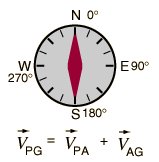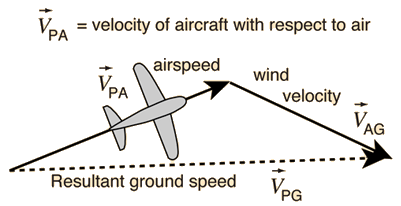This calculation is a straighforward vector addition of the airplane's airspeed and the wind velocity.

Velocity unit
 For airspeed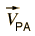= at °
 and wind speed= at °
 the resultant ground velocity is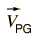= at °

Note: The wind direction in this calculation is the direction of air motion, not the direction from which the wind is coming. So if you call a wind from the north a "north wind", then the air motion direction is south and you would enter 180° for the wind angle.

 Alternate calculations: Wind velocity Desired heading and resultant
Index

Relative velocity

 HyperPhysics***** Mechanics R Nave
Go Back

# Discussion of Airplane in WindNavigation directions are usually expressed in terms of compass angles as illustrated. The vector addition necessary to calculate resultant velocity is carried out by calculating the components of each vector.There are some practical problems associated with this calculation: you can either convert compass angles to standard angles, or do the calculation in compass angles with the relationships shown. If you calculate the standard angle for the final bearing, it can then be converted to compass angle. A final problem is the arctan problem with calculators and computer languages: you have to check the quadrant to be sure you get the correct angle.

### Calculation

Index

Relative velocity

 HyperPhysics***** Mechanics R Nave
Go Back